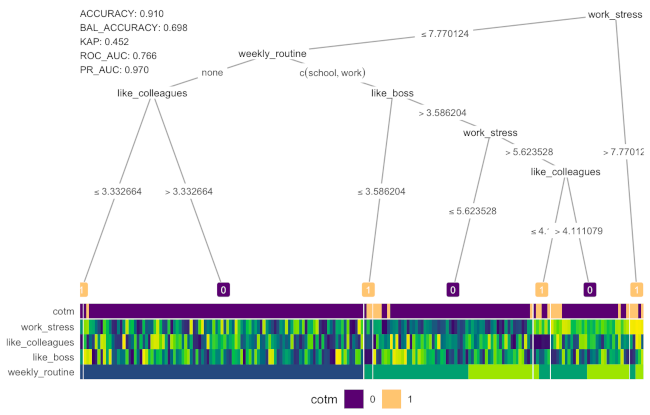September 15, 2020

## Overview

1. Background

2. Basic Implementation

Dataset & research problem; Model basics & key terms (OOB error & parameters); Tuning; Prediction; Variable Importance

3. Gotchas

Imbalanced samples; Variable importance validation; Multicollinearity

## Trees vs Forests

Decision Tree Random Forest
Single tree Collection of different trees
Uses entire dataset Uses boostrapped data
Uses all features Uses subsets of features
One tree determines prediction All trees contribute to prediction

## Random Forest Algorithm

The basic algorithm for a regression random forest can be generalized to the following: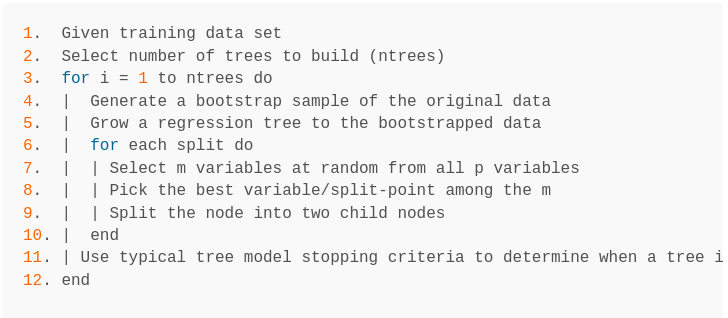(from UC Business Analytics R Programming Guide)

Good performance Advanced boosting algorithms can perform better
“out-of-the box” Slow on large data sets
Built-in validation set Not very interpretable
Robust to outliers

## The Case of the Mondays (COTM)## Dataset

Ficticious scientists conducted a ficticious survey of 1000 ficticious people, collecting the following variables of interest:

• Age
• Weekly routine (does the person have a regular routine of school/work or not?)
• How much fun is had on weekends
• Alcohol consumption on weekends
• How much work, boss and colleagues are liked
• How much work stress is experienced
• Health, Financial and Social support status

## Train & Test Samples

set.seed(123)   # for reproduciblity

train <- sample(1:nrow(df), .67*nrow(df))

df_train <- df[train,]
df_test <- df[-train,]## Model

# library(randomForest)
set.seed(123)   # for reproduciblity

rf <- randomForest(formula =  cotm ~ ., data  = df_train,
importance = TRUE)
rf
##
## Call:
##  randomForest(formula = cotm ~ ., data = df_train, importance = TRUE)
##                Type of random forest: classification
##                      Number of trees: 500
## No. of variables tried at each split: 3
##
##         OOB estimate of  error rate: 8.36%
## Confusion matrix:
##     0  1 class.error
## 0 601  7  0.01151316
## 1  49 13  0.79032258

## Model Parameters

set.seed(123)   # for reproduciblity

rf_formula = paste0("cotm ~ ",
paste0(colnames(df[,-2]), collapse = " + "))

rf1 <- randomForest(formula =  as.formula(rf_formula),
data  = df_train,
ntree = 300,   # number of trees
mtry = 2,      # number of vars sampled per split
sampsize = 400, # size of samples to draw
nodesize = 3, # minimum number of terminal nodes
importance = TRUE)   # keep importance values of variables

## Tuning

# library(ranger)

# hyperparameter grid search
hyper_grid <- expand.grid(
num_trees = seq(400,600, by = 50),
mtry       = seq(2, 11, by = 2),
node_size  = seq(3, 9, by = 2),
sample_size = c(.55, .632, .70, .80),
OOB_RMSE   = 0
)

Total number of combinations: 400

## Tuning

for(i in 1:nrow(hyper_grid)) {

# train model
model <- ranger(
formula         = cotm ~ .,
data            = df_train,
num.trees       = hyper_grid$num_trees[i], mtry = hyper_grid$mtry[i],
min.node.size   = hyper_grid$node_size[i], sample.fraction = hyper_grid$sample_size[i],
seed            = 123
)

# add OOB error to grid
hyper_grid$OOB_RMSE[i] <- sqrt(model$prediction.error)
}

## Tuning

hyper_grid <- hyper_grid %>%
dplyr::arrange(OOB_RMSE)

head(hyper_grid, 5)
##   num_trees mtry node_size sample_size  OOB_RMSE
## 1       400    8         9        0.55 0.2676598
## 2       450    8         9        0.55 0.2704336
## 3       550    6         9        0.55 0.2731792
## 4       400   10         9        0.55 0.2731792
## 5       600   10         9        0.70 0.2731792

## Tuning

set.seed(123)
rf2 <- randomForest(formula = as.formula(rf_formula), data  = df_train,
ntree = hyper_grid$num_trees, mtry = hyper_grid$mtry,
sampsize = ceiling(hyper_grid$sample_size*nrow(df)), nodesize = hyper_grid$node_size, importance = TRUE)
rf2
##
## Call:
##  randomForest(formula = as.formula(rf_formula), data = df_train,      ntree = hyper_grid$num_trees, mtry = hyper_grid$mtry,      sampsize = ceiling(hyper_grid$sample_size * nrow(df)), nodesize = hyper_grid$node_size, importance = TRUE)
##                Type of random forest: classification
##                      Number of trees: 400
## No. of variables tried at each split: 8
##
##         OOB estimate of  error rate: 7.46%
## Confusion matrix:
##     0  1 class.error
## 0 600  8  0.01315789
## 1  42 20  0.67741935

## Prediction

predicted_values <- predict(rf2, df_test)
confusionMatrix(predicted_values, df_test$cotm)$table
##           Reference
## Prediction   0   1
##          0 290  25
##          1   2  13

## Variable importance: Accuracy

# For each variable, scramble values (maintain distribution); build a tree;
# assess accuracy and loss of accuracy as a result of scrambling.
varImpPlot(rf2, type = 1)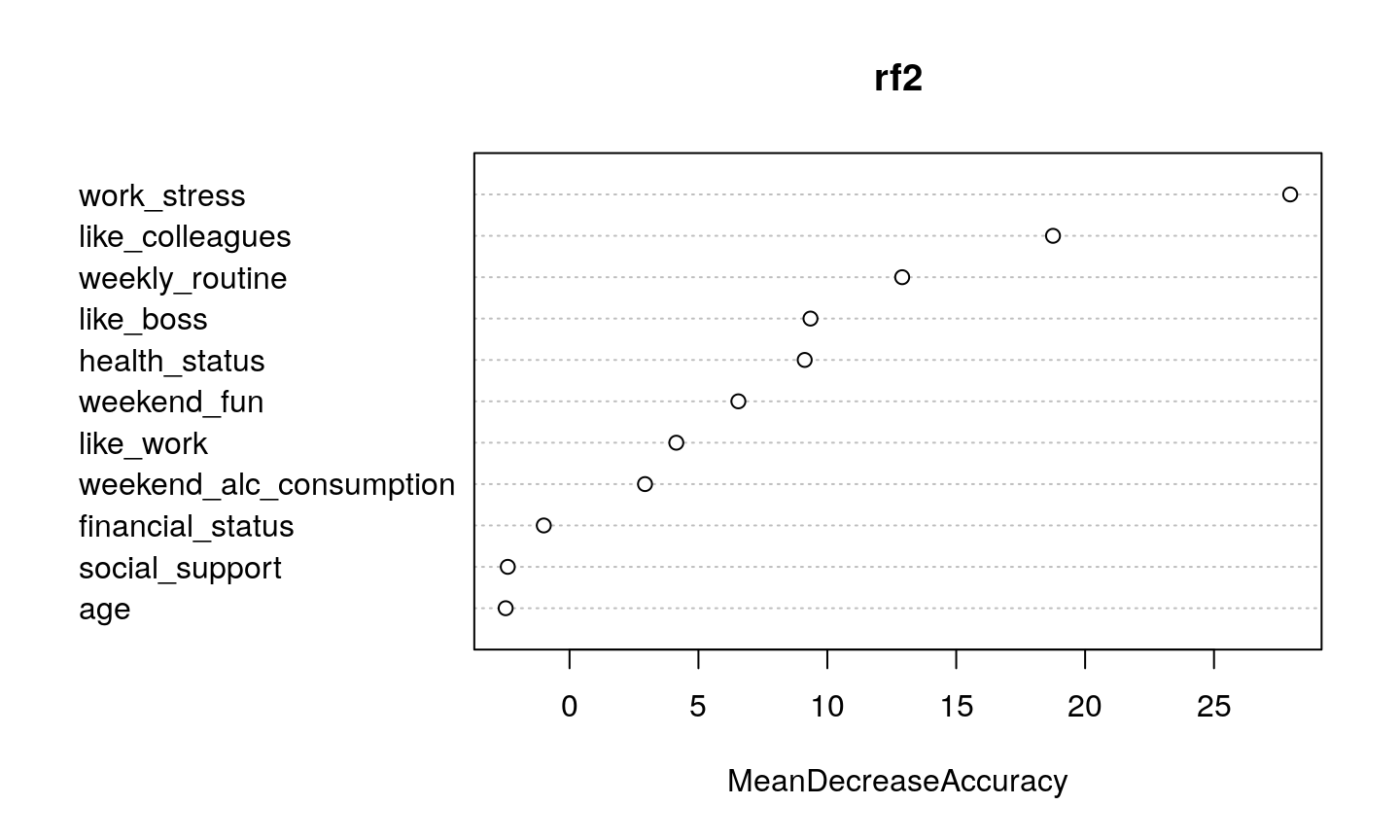## Variable importance: Gini

# If the variable is useful, it will split mixed labeled nodes into
# pure single class nodes. Gini index is an indicator of node 'purity'.
varImpPlot(rf2, type = 2)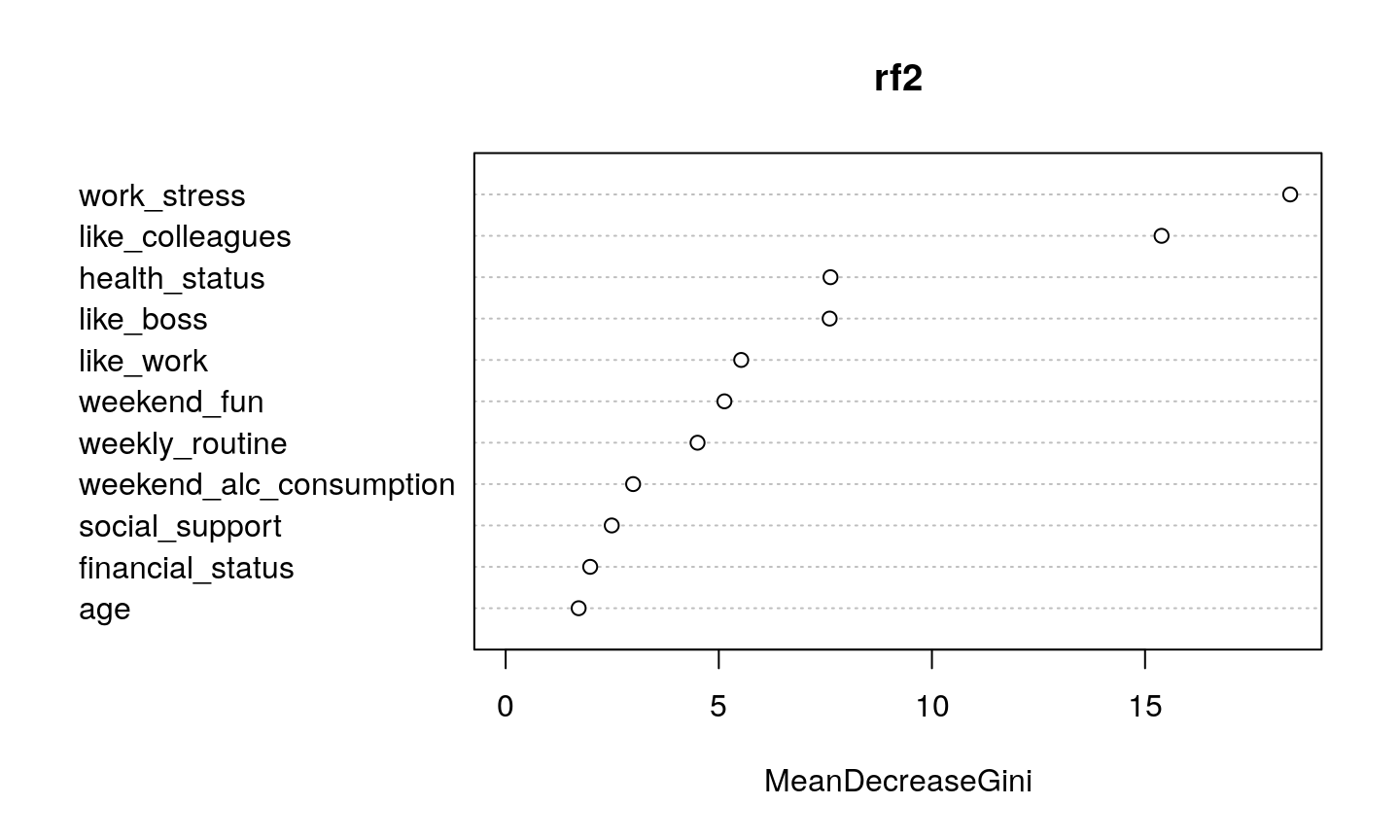## Imbalanced samples

set.seed(123)
rf <- randomForest(formula =  cotm ~ ., data  = df_train_balanced)
rf
##
## Call:
##  randomForest(formula = cotm ~ ., data = df_train_balanced)
##                Type of random forest: classification
##                      Number of trees: 500
## No. of variables tried at each split: 3
##
##         OOB estimate of  error rate: 17.74%
## Confusion matrix:
##    0  1 class.error
## 0 51 11   0.1774194
## 1 11 51   0.1774194

## Some solutions

(see this tech report for additional info)

1. Balance data via under/over sampling
2. Add weights to random forest model
3. Quantile-classifer (RFQ) approach

Package randomForestSRC has implementations for 2 & 3

## Validation of variable importance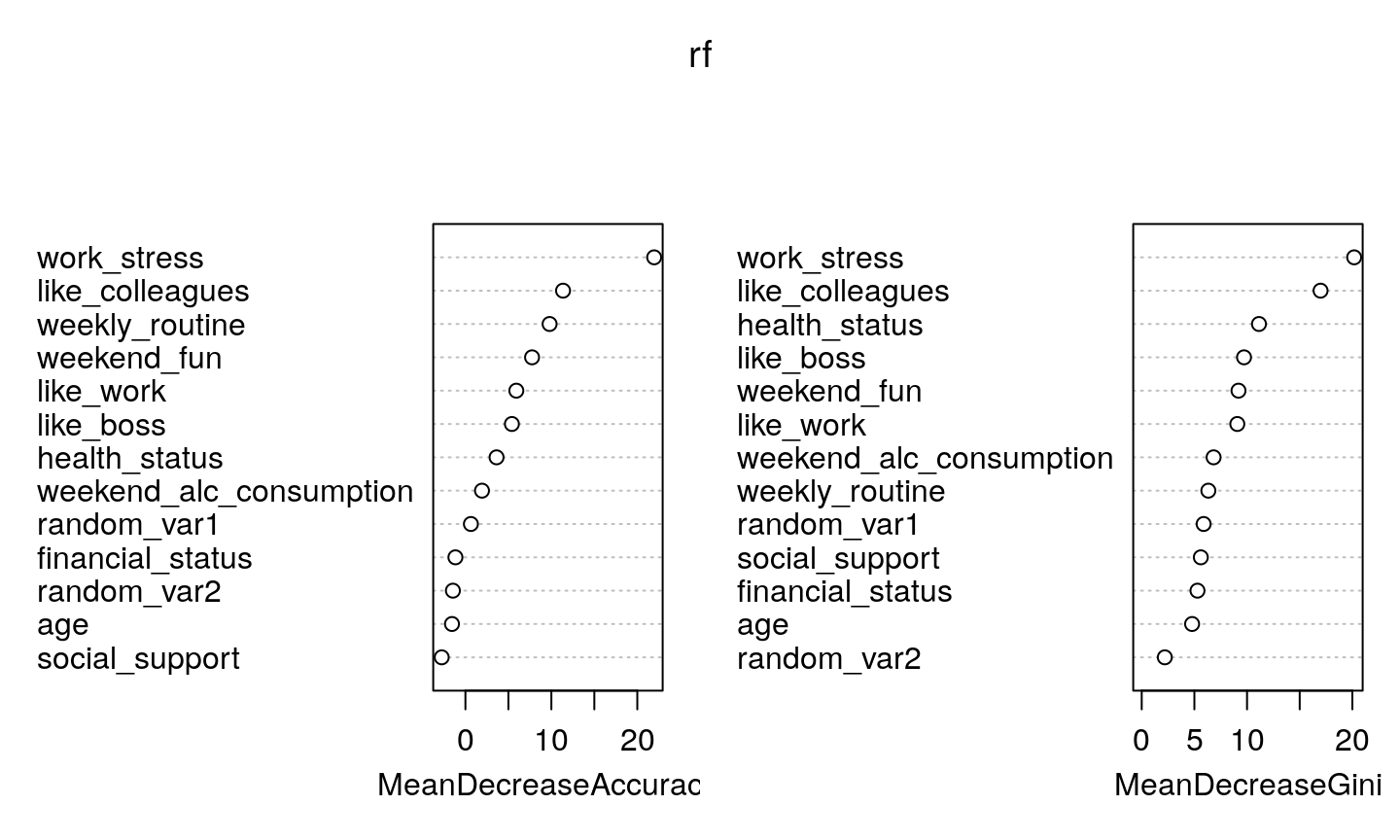## Correlated predictors

Random forests can be sensitive to correlated predictors; this is particularly problematic when using random forest for variable selection. Some alternatives:

1. Conditional Inference Forests (partykit::cforest)
2. Boruta (Boruta::Boruta)

## Case of the Mondays, via treeheatr!

Check out treeheatr here!